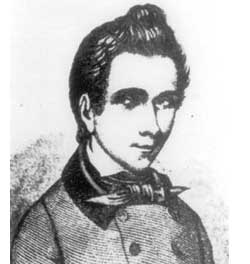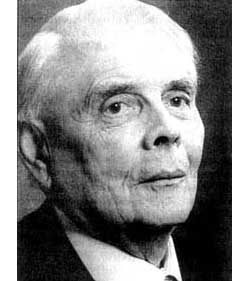Even if you don’t know the formal definition of a profinte group, you know at least one example which explains the concept : the Galois group of the algebraic numbers $Gal = Gal(\overline{\mathbb{Q}}/\mathbb{Q})$ aka the absolute Galois group. By definition it is the group of all $\mathbb{Q}$-isomorphisms of the algebraic closure $\overline{\mathbb{Q}}$. Clearly, it is an object of fundamental importance for mathematics but in spite of this very little is known about it. For example, it obviously is an infinite group but, apart from the complex conjugation, try to give one (1!) other nontrivial element… On the other hand we know lots of finite quotients of $Gal$. For, take any finite Galois extension $\mathbb{Q} \subset K$, then its Galois group $G_K = Gal(K/\mathbb{Q})$ is a finite group and there is a natural onto morphism $\pi_K~:~Gal \rightarrow G_K$ obtained by dividing out all $K$-automorphisms of $\overline{\mathbb{Q}}$. Moreover, all these projections fit together nicely. If we take a larger Galois extension $K \subset L$ then classical Galois theory tells us that there is a projection $\pi_{LK}~:~G_L \rightarrow G_K$ by dividing out the normal subgroup of all $K$-automorphisms of $L$ and these finite maps are compatible with those from the absolute Galois group, that is, for all such finite Galois extensions, the diagram below is commutative

$$\xymatrix{Gal \ar[rr]^{\pi_L} \ar[rd]_{\pi_K} & & G_L \ar[ld]^{\pi_{LK}} \\ & G_K &}$$

By going to larger and larger finite Galois extensions $L$ we get closer and closer to the algebraic closure $\overline{Q}$ and hence a better and better finite approximation $G_L$ of the absolute Galois group $Gal$. Still with me? Congratulations, you just rediscovered the notion of a profinite group! Indeed, the Galois group is the projective limit

$Gal = \underset{\leftarrow}{lim}~G_L$

over all finite Galois extensions $L/\mathbb{Q}$. If the term ‘projective limit’ scares you off, it just means that all the projections $\pi_{KL}$ coming from finite Galois theory are compatible with those coming from the big Galois group as before. That’s it : profinite groups are just projective limits of finite groups.These groups come equipped with a natural topology : the Krull topology. Again, this notion is best clarified by considering the absolute Galois group. Now that we have $Gal$ we would like to extend the classical Galois correspondence between subgroups and subfields $\mathbb{Q} \subset K \subset \overline{\mathbb{Q}}$ and between normal subgroups and Galois subfields. For each finite Galois extension $K/\mathbb{Q}$ we have a normal subgroup of finite index, the kernel $U_K=Ker(\pi_K)$ of the projection map above. Let us take the set of all $U_K$ as a fundamental system of neighborhoods of the identity element in $Gal$. This defines a topology on $Gal$ and this is the Krull topology. As every open subgroup has finite index it is clear that this turns $Gal$ into a compact topological group. Its purpose is that we can now extend the finite Galois correspondence to Krull’s Galois theorem :

There is a bijective lattice inverting Galois correspondence between the set of all closed subgroups of $Gal$ and the set of all subfields $\mathbb{Q} \subset F \subset \overline{\mathbb{Q}}$. Finite field extensions correspond in this bijection to open subgroups and the usual normal subgroup and factor group correspondences hold!

So far we had a mysterious group such as $Gal$ and reconstructed it from all its finite quotients as a projective limit. Now we can reverse the situation : suppose we have a wellknown group such as the modular group $\Gamma = PSL_2(\mathbb{Z})$, then we can look at the set of all its normal subgroups $U$ of finite index. For each of those we have a quotient map to a finite group $\pi_U~:~\Gamma \rightarrow G_U$ and clearly if $U \subset V$ we have a quotient map of finite groups $\pi_{UV}~:~G_U \rightarrow G_V$ compatible with the quotient maps from $\Gamma$

$$\xymatrix{\Gamma \ar[rr]^{\pi_U} \ar[rd]_{\pi_V} & & G_U \ar[ld]^{\pi_{UV}} \\ & G_V &}$$

For the family of finite groups $G_U$ and groupmorphisms $\pi_{UV}$ we can ask for the ‘best’ group mapping to each of the $G_U$ compatible with the groupmaps $G_{UV}$. By ‘best’ we mean that any other group with this property will have a morphism to the best-one such that all quotient maps are compatible. This ‘best-one’ is called the projective limit

$\hat{\Gamma} = \underset{\leftarrow}{lim}~G_U$

and as a profinite group it has again a Krull topology making it into a compact group. Because the modular group $\Gamma$ had quotient maps to all the $G_U$ we know that there must be a groupmorphism to the best-one
$\phi~:~\Gamma \rightarrow \hat{\Gamma}$ and therefore we call $\hat{\Gamma}$ the profinite compactification (or profinite completion) of the modular group.

A final remark about finite dimensional representations. Every continuous complex representation of a profinite group like the absolute Galois group $Gal \rightarrow GL_n(\mathbb{C})$ has finite image and this is why they are of little use for people studying the Galois group as it conjecturally reduces the study of these representations to ‘just’ all representations of all finite groups. Instead they consider representations to other topological fields such as p-adic numbers $Gal \rightarrow GL_n(\mathbb{Q}_p)$ and call these Galois representations.

For people interested in Grothendieck’s dessins d’enfants, however, continuous complex representations of the profinite compactification $\hat{\Gamma}$ is exactly their object of study and via the universal map $\phi~:~\Gamma \rightarrow \hat{\Gamma}$ above we have an embedding

$\mathbf{rep}_c~\hat{\Gamma} \rightarrow \mathbf{rep}~\Gamma$

of them in all finite dimensional representations of the modular group (
and we have a similar map restricted to simple representations). I hope this clarifies a bit obscure terms in the previous post. If not, drop a comment.

Published in featured